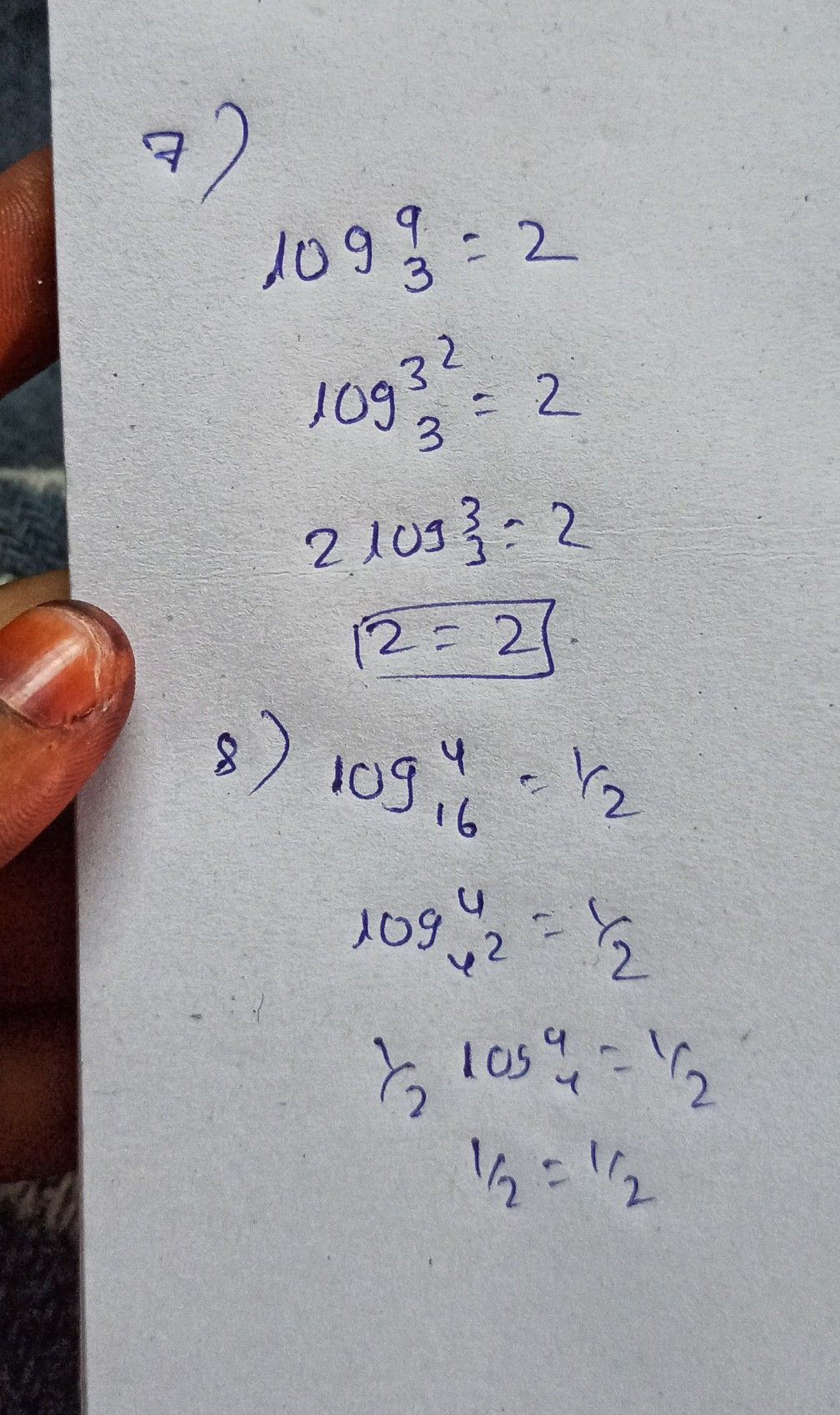Symbol
ProblemActivity 1. $0$ $cc$ $02$ In numbers 1-3, find the value of the following logarithmic expressions. 1. $log _{3}81$ 2. $log _{169}13$ $3.log _{s}\dfrac {1} {5}$ In numbers $4-6$ rewrite the following expressions in logarithmic form, whenever possible. $4.16$ $16=2^{4}$ $5.9=\sqrt{81}$ $6.\dfrac {1} {9}=3-2$ In number 7- $-9$ rewrite the following logarithmic equations in exponential $tom$ whenever $possiblc$ $7.log _{3}9=2$ 8. $log _{16}4=\dfrac {1} {2}$ $9.lnx=1$ 10. What is the magnitude in the Richter scale of an earthquake that released $10^{14}$ joules of energy? How much more energy does this earthquake release than that of the reference earthquake? $1a$
10th-13th grade
Other
Search count: 137
SolutionQanda teacher - vyshnavi